Next: References Up: The derivation of the Previous: The Derivation

Linear observations

Upon reflection of equations (11) and (12) a problem arises: the determination of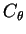and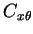require having the true field values, x, but all one can actually observe are the measurement data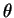.

In order to account for this important distinction we need to make some assumptions about how the measurements are related to the actual state of the system. We will assume that the observations are a linear function of the actual state plus random noise,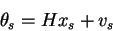(13)

where H is a known matrix that maps the data to the observations and v is the random measurement error or noise. We introduce the index s here in order to be explicit that we are indicating the values at some space-time location s, where the observations are, which is not necessarily the location where the estimate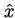of the field is being made.

With this, we can write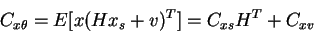(14)

Applying (13) to the definition of, gives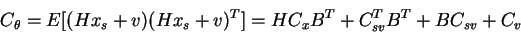(15)

If we suppose that the actual state and the noise are uncorrelated, then the terms, Cxv and Csv are each zero.

So now we have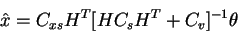(16)

and,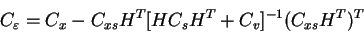(17)

If we consider the case where the measurementsare the same quantity as what we are estimating x (i.e. we are using density data to estimate the density field, as opposed to using salinity and temperature to estimate the density field), then H is just the identity matrix, so our estimator is,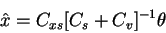(18)

and,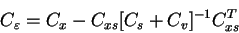(19)

If the noise is white noise, then Cv is a diagonal matrix and we see that the effect of not having the true state correlations, but estimates of it based upon the observations, is to increase the diagonal elements of the matrix to be inverted by the measurement noise variance.Next: References Up: The derivation of the Previous: The Derivation
Skip Carter
1999-12-08